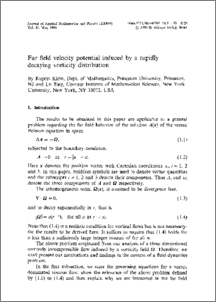Repository: Freie Universität Berlin, Math Department

# Far field velocity potential induced by a rapidly decaying vorticity distribution

Klein, R. and Ting, L. (1990) Far field velocity potential induced by a rapidly decaying vorticity distribution. Zeitschrift für Angewandte Mathematik und Physik (ZAMP), 41 (3). pp. 395-418. ISSN 0044-2275 (Print) 1420-9039 (Online)Preview

1MB

We study the velocity field induced by a vorticity distribution decaying rapidly in the distancer from the origin. In the far field, the vector potential for the velocity field can be represented by a series SgrA (n), withA (n) proportional tor –n–1, forn=1, 2, .... We show thatA (n) can be expressed as a linear combination ofM n linearly independent vector functions. The numberM n is equal to 3 forn=1 and 4n fornge2 and the coefficient of a vector function is defined by a linear combination of $$\frac{3}{2}(n + 1)(n + 2)$$ nth moments of vorticity. We then show that only 2n+1 linear combinations of thoseM n vector functions contribute to the far field velocity which is irrotational. The corresponding scalar potential PHgr (n) is then represented by a linear combination of 2n+1 spherical harmonics ofnth order whose coefficients are again linear combinations ofnth moments of vorticity.